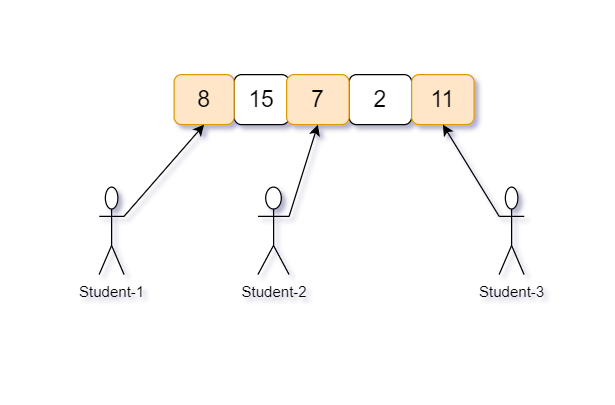Chocolate Problem

#### Example :

``````Given 'N' : 5 (number of packets) and 'M' : 3 (number of students)
````````````And chocolates in each packet is : {8, 11, 7, 15, 2}

All possible way to distribute 5 packets of chocolates among 3 students are -

( 8,15, 7 ) difference of maximum-minimum is ‘15 - 7’ = ‘8’
( 8, 15, 2 ) difference of maximum-minimum is ‘15 - 2’ = ‘13’
( 8, 15, 11 ) difference of maximum-minimum is ‘15 - 8’ = ‘7’
( 8, 7, 2 ) difference of maximum-minimum is ‘8 - 2’ = ‘6’
( 8, 7, 11 ) difference of maximum-minimum is ‘11 - 7’ = ‘4’
( 8, 2, 11 ) difference of maximum-minimum is ‘11 - 2’ = ‘9’
( 15, 7, 2 ) difference of maximum-minimum is ‘15 - 2’ = 13’
( 15, 7, 11 ) difference of maximum-minimum is ‘15 - 7’ = ‘8’
( 15, 2, 11 ) difference of maximum-minimum is ‘15 - 2’ = ‘13’
( 7, 2, 11 ) difference of maximum-minimum is ‘11 - 2’ = ‘9’

Hence there are 10 possible ways to distribute ‘5’ packets of chocolate among the ‘3’ students and difference of combination (8, 7, 11) is ‘maximum - minimum’ = ‘11 - 7’ = ‘4’ is minimum in all of the above.
``````
##### Input format :
``````The first line of input contains an integer ‘T’ denoting the number of test cases.
The next ‘2*T’ lines represent the ‘T’ test cases.

The first line of each test case contains two space-separated integers ‘N’ denoting the number of packets of chocolate and ‘M’ denotes the number of students.

The second line of each test case contains ‘N’ space-separated integers denoting the number of chocolate in each of ‘N’ packets.
``````
##### Output Format :
``````For each test case, print the minimum difference of the chocolates contained in the packets distributed to the students.
``````
##### Note :
``````You don't need to print anything, it has already been taken care of. Just implement the given function.
``````
##### Constraints:
``````1 <= T <= 50
2 <= M <= N <= 10^4
1 <= CHOCOLATES[i] <= 10^9

Time Limit : 1 sec
``````
#TataConsultancyServices(Tcs)#AssociateSoftwareEngineerCodingNinjas
Answered Mar 09, 2022
Recursion

The idea is that generate all the possible subsets of size ‘M’ and checks the minimum difference that can be possible from all subsets.

1. This problem can be solved using recursion and the idea behind this is to generate all the possible minimum differences from the given array.
2. To store minimum difference we use a variable (say, ‘minVal’) and the initial value is ‘INFINITE’
3. For each element we will have two choices: either to include it in the first subset or not. So, at each stage, we will make two recursive calls, one which will consider including the current element and others which won’t. We will explore the depth taking into consideration each of the two possible cases.
4. When we have no elements to explore we will then check our condition on a particular set that can make a minimum difference.
5. Suppose we are at set ‘S’ and the size of ‘S’ is ‘M’ then find a ‘minimum’ and ‘maximum’ value of set ‘S’ and compare is 'maximum' - ‘minimum’ is less than ‘minVal’
• If yes then update ‘minVal’ with ‘maximum’ - ‘minimum’
6. Finally, return ‘minVal’
Space Complexity: O(n)Explanation:

O(N), where ‘N’ is the number of elements in the array.

This space is used to store the recursion stack. Our algorithm works in a depth - first way, so we cannot have more than ‘N’ recursive calls on the stack as the total numbers present can be at max ‘N’. Hence, the overall space complexity will be O(N).

Time Complexity: O(2^n)Explanation:

O(2 ^ N), where ‘N’ is the number of integers in the array.

Each number has two choices, whether to include it in a set or not. There are total ‘N’ numbers present with us, so the total possible combinations will be 2 * 2 * 2… (‘N’ times). Hence, the overall time complexity will be O(2 ^ N).

ReportCodingNinjas
Answered Mar 09, 2022
Sliding Window

The idea is based on the observation that to minimize the difference between a maximum number of chocolate in a packet and a minimum number of chocolate in the packet is that, always choose consecutive packets of chocolate in sorted order of a number of chocolates in a packet. In the below figure it is clear that the minimum difference can get by selecting consecutive packets of chocolate that is ( 1, 4, 7 ), and the difference is ‘7 - 1’ = ‘6’

Here is the algorithm :

1. Suppose the given array is ‘CHOCOLATES’.
2. Sort the given array.
3. Find the subarray with the minimum difference of the last and first elements.
4. To store minimum difference we use a variable (say, ‘minVal’) and the initial value is ‘INFINITE’
5. Run a loop from 0 to ‘N’ - ‘M’ - 1(say, iterator ‘i’)
6. If 'CHOCOLATES'[i] - ‘CHOCOLATES’[i - M + 1] is less than ‘minVal’ then update ‘minVal’ with ‘CHOCOLATES’[i] - ‘CHOCOLATES’[i - M + 1] because we can get minimum difference possible with ‘i’th and (’i' - ‘M’ + 1)’th packet.
7. Finally, return ‘minVal’
Space Complexity: O(1)Explanation:

O(1)

We are using no extra space. Hence, the overall space complexity will be O(1).

Time Complexity: O(nlogn)Explanation:

O(N * log(N)), Where ‘N’ is the number of packets of chocolates.

We are sort the ‘CHOCOLATES’ to find the minimum difference possible which have O(N * log(N)) complexity and iterate through the array. Hence, the overall time complexity will be O(N * log(N)).

Report